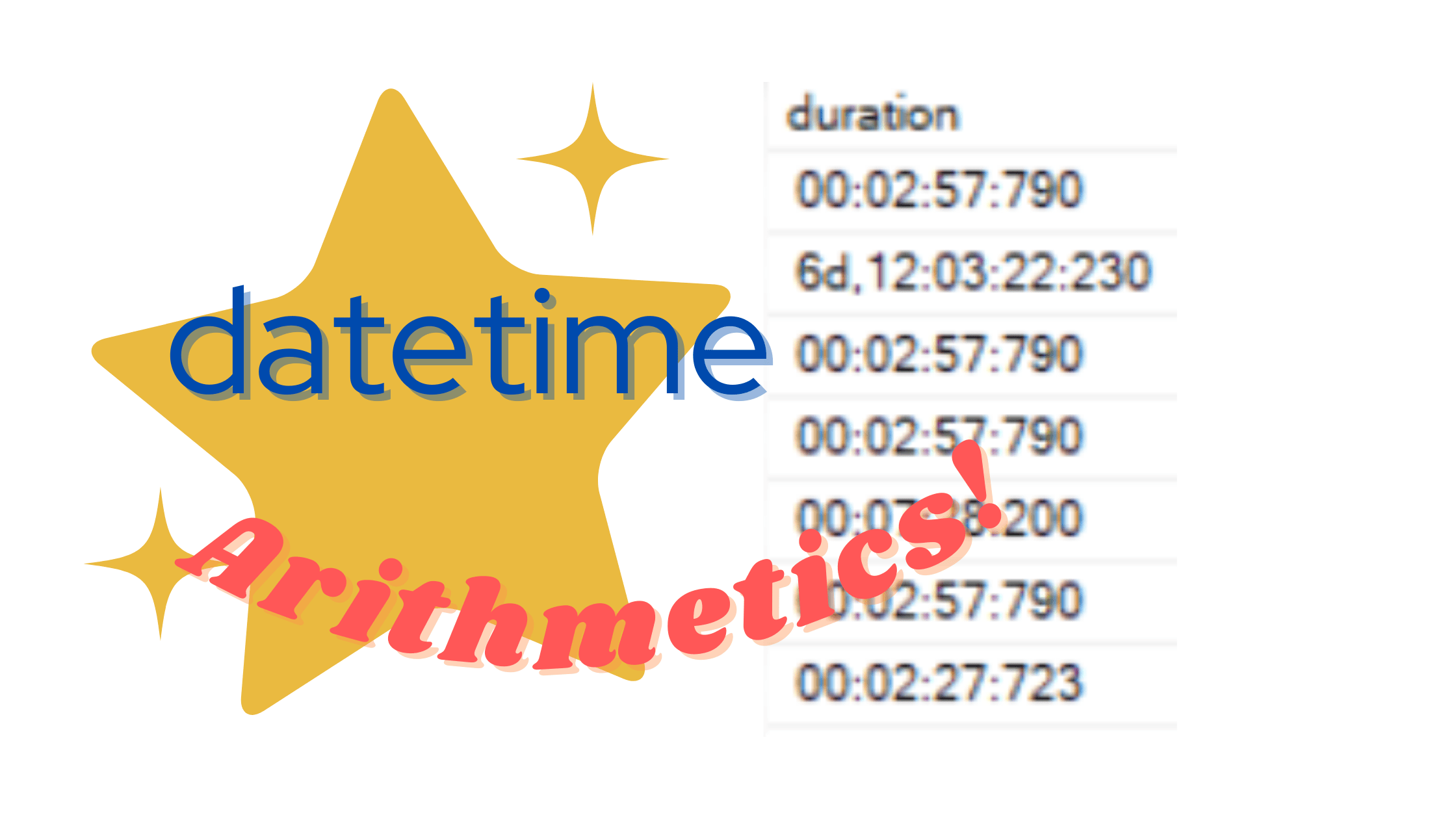Home » Even more fun with DATETIME arithmetics!

# Even more fun with DATETIME arithmetics!

In one of my previous posts, Fun with DATETIME Arithmetics, I introduced a way to use “math” to manipulate `datetime` values for effectively generating, calculating, and displaying intervals (i.e. difference between two `datetime` values). These mostly work with the addition and subtraction operators (+, -).

In one of the paragraphs, I mentioned multiplication and division, and posed the question about why anyone would ever need to do this.

Reminder:

## Any plans for DATEMULTIPLY and DATEDIVIDE?

Multiplication (*) and division (/) won’t work:

```SET @d2 = @d1 * 2.0
```
`Msg 257, Level 16, State 3, Line 21Implicit conversion from data type datetime to numeric is not allowed. Use the CONVERT function to run this query.`

But it’s possible if you convert the datetime values to `float `first (although I’m not entirely sure why you would ever need to do something like this):

```SET @d2 = CONVERT(float, @d1) * 2.0

SELECT @d2 -- result: 1900-01-04 08:30:31.800

SELECT CONVERT(datetime, CONVERT(float, @d1) * CONVERT(float, @d2))
-- result: 1900-01-06 15:02:05.420
```

Well, it just so happens that I have recently stumbled upon a use-case which could in fact be easily solved using multiplication and division on `datetime` values.

## Tell me if this looks familiar

Have you ever heard of the use case where one would have need to “round” a `datetime` value down to a certain date-part, such as a day, month, hour, minute, etc.?

For example, assuming that we have a datetime value such as `'2021-06-12 12:34:56.456'`, and we want to “round” it down to:

```nearest month -> '2021-06-01 00:00:00.000'
nearest day -> '2021-06-12 00:00:00.000'
nearest hour -> '2021-06-12 12:00:00.000'
nearest minute -> '2021-06-12 12:34:00.000'
nearest second -> '2021-06-12 12:34:56.000'```

You get the idea, right?

If you wanna do that, there’s an old-timey method to do so using a combination of DATEADD and DATEDIFF functions. For example, here is how to round a `datetime` value down to the nearest day:

```DATEADD(dd, DATEDIFF(dd, 0, MyDateTimeValue), 0)
```

The general “algorithm” is this:

1. Calculate how many intervals of a specific date-part (e.g. days) are between your `datetime `value, and the “zeroth” datetime (i.e. “0”):
`DATEDIFF(dd, 0, MyDateTimeValue)`
2. Add that number of intervals to the “zeroth” datetime:
`DATEADD(dd, ..., 0)`
3. Any part of your `datetime `value which is smaller than the specified date-part will be ignored / truncated.

Simply replace the “dd” date-part with any other date-part as needed, and it would do the job well.

## But when is it not good enough?

The method above is very useful and easy to implement… As long as you need to round your `datetime `value to something that exists as a supported date-part in the DATEADD and DATEDIFF functions.

But… What if you have an unusual use-case, where you need the rounding to be down to something which does NOT exist as a built-in date-part?

For example, what if you need your `datetime` value to be rounded down to the nearest 10 minutes? Or 5 minutes? Or 30 minutes? Or 3 days? There is no date-part for that, buddy.

## Time to Multiply your Divisions

But what we DO have is (as you probably have guessed) datetime arithmetics!

Assuming that we can represent our desired interval as a `datetime` value (for example, 10 minutes would be: `'00:10:00'`), we can plug it into the same “algorithm” that we implemented using the aforementioned DATEADD+DATEDIFF method, but this time make it work with a datetime-typed interval.

It would look something like this:

```CONVERT(datetime,
FLOOR(CONVERT(float, @MyDateTimeValue) / CONVERT(float, @interval))
* CONVERT(float, @interval)
)
```

The “algorithm” is essentially the same:

1. Calculate how many intervals are between your datetime value and the “zeroth” datetime, which would be the same as:
`FLOOR(CONVERT(float, @MyDateTimeValue) / CONVERT(float, @interval))`
2. Add that number of intervals to the “zeroth” datetime, which is the same as:
`CONVERT(datetime, ... * CONVERT(float, @interval) )`

In other words:

1. We convert both the `datetime` value and the desired `interval` to `float`. This would already represent the difference in days between the given `datetime` and the “zeroth” `datetime` (“0”).
2. We find how many times the desired interval “fits” into the `datetime` value (using division).
3. We activate the FLOOR function on that result so that anything “smaller” than the desired interval would be ignored / truncated. Now our result is basically something like “how many 10 minutes are in my datetime”.
4. Take that result and multiply it by our desired `interval`, to get our new `datetime` value. But wait, it’s actually still `float`. So:
5. Convert the result back to `datetime`.

Here is an example script:

```DECLARE @MyDateTimeValue DATETIME = '2021-06-12 12:37:54', @interval DATETIME = '00:10:00'

SELECT CONVERT(datetime,
FLOOR(CONVERT(float, @MyDateTimeValue) / CONVERT(float, @interval))
* CONVERT(float, @interval)
)
```

You can play around with the variables `@MyDateTimeValue` and `@interval` and see that this method works well.

Additionally, by simply replacing the FLOOR function here with its opposite function CEILING, you can very easily round your `datetime` value UP instead of DOWN:

```DECLARE @MyDateTimeValue DATETIME = '2021-06-12 12:37:54', @interval DATETIME = '00:10:00'

SELECT CONVERT(datetime,
CEILING(CONVERT(float, @MyDateTimeValue) / CONVERT(float, @interval))
* CONVERT(float, @interval)
)
```

Play around with this too for a bit to see how this works.

## Conclusion

Obviously, using the “vanilla” functions DATEADD and DATEDIFF would be more readable and preferable where possible.

But once in a while, you would come across an unusual requirement that would call for more “creative” solutions.

For these unusual circumstances, I hope you’d find this post here useful 😊.

## 1 thought on “Even more fun with DATETIME arithmetics!”

This site uses Akismet to reduce spam. Learn how your comment data is processed.